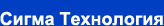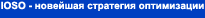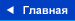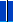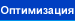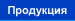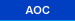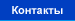### Многокритериальная оптимизация

Алгоритмы IOSO технологии позволяют успешно решать задачи многокритериальной (десятки критериев) многомерной (сотни переменных) нелинейной оптимизации. Основные достоинства наших алгоритмов заключаются в том, что:
• не используются сверточные подходы при решении многокритериальных задач;
• определяется желаемое количество Парето-оптимальных решений, равномерно распределенных в пространстве критериев;
• возможно решение задач для целевых функций со сложной топологией (невыпуклые, недифференцируемые, многоэкстремальные);
• естественным образом используется распараллеливание вычислительного процесса.

Наши алгоритмы многокритериальной оптимизации являются незаменимым инструментом при решении задач многодисциплинарной и многоуровневой оптимизации, а также для оптимизации при наличии неопределенностей.

##### Формулировка проблемы

Most real-life engineering optimization problems require the simultaneous optimization of more than one objective function. In these cases, it is unlikely that the different objectives would be optimized by the same alternative parameter choices. Hence, some trade-off between the criteria is needed to ensure a satisfactory design.

As the system efficiency indices can be different (and mutually contradictory), it is reasonable to use the multicriteria approach to optimize the overall efficiency.

This can be done mathematically correctly only when some optimality principle is used. We use Pareto optimality principle, the essence of which is following. The multicriteria optimization problem solution is considered to be Pareto-optimal if there are no other solutions that are better in satisfying all of the objectives simultaneously. That is, there can be other solutions that are better in satisfying one or several objectives, but they must be worse than the Pareto-optimal solution in satisfying the remaining objectives.

In this case multicriteria optimization problem consists in a full set of Pareto optimal solutions determination.

As a rule, it is impossible to find the full infinite set of Pareto optimal solutions under the real problems solving. For this reason the engineering multicriteria problem statement consists in determination of the finite subset of criteria-distinguishable Pareto optimal solutions.

##### Наш метод

Preliminary procedure of our optimization method consists of the initial plan of experiment forming (XW). For each vector of variables, the values of the optimization criteria and constraints are evaluated using the mathematical model of the system under investigation.

The basic optimization algorithm may be presented schematically as a following sequence of steps.

Step 1. From the given plan of experiment all of the distinguishable Pareto optimal points (subset A) are extracted (the number of this points is n>=1).

Step 2. From the current Pareto optimal points (subset A) the single point xcur is randomly selected.

Step 3. The subset of the plan of experiment XM is constructed. XM consists of M points, closest to the current point xcur according to the linear metric. The current search area is defined.

Step 4. Within the current area of search the response surfaces of the particular optimization objectives are constructed.

Step 5. Within the current search area the optimization tasks are solved using previously constructed response surfaces. As a result of this step the number of points are identified that are suspected to be the particular objectives extremums for the current search area.

Step 6. At these points the true value of the particular objectives are evaluated using the mathematical model of the system under investigation. The plan of experiment is modified.

Step 7. The stop criterion is checked.

Step 8-a. GO TO Step 1.

Step 8-b. In the vicinity of the current Pareto optimal point the additional points are generated using the normal distribution. These points are included as the components of the plan of experiment, GO TO Step 1.

It is vital to note, that at the initial stage of optimization process the accuracy of the response surfaces may be not so good due to the small number of points in the plan of experiment and the relatively large size of the current search area. However, during the optimization process, the number of points in the vicinity of the Pareto optimal points, is increased. At the same time, the size of the current search area is decreased. These trends are leading to a more accurate approximation of the objective functions and, hence, to the optimization process efficiency increase. Actually, during the optimization process, the information about the objective functions in the vicinity of the Pareto optimal points is permanently accumulated.

A number of heuristic procedures are developed for proposed optimization method efficiency increase as the information accumulates. These procedures are directed towards the adaptive change in the numbers of points in the plan of experiment and in the current search area. The adaptations affect the value of the normal distribution parameter and the rational selection of the step 8-a or the step 8-b in the basic optimization algorithm.

The main advantages of the proposed indirect multicriteria optimization method over traditional mathematical programming strategies lie in the possibility of the problem solving in case of nonconvex, discontinuous and stochastic goal functions and constraints; the unnecessity of considerable adaptation of the mathematical model for the optimization problem solving; the possibility to obtain a set of EP-optimal decisions under relatively small number of turns to the mathematical model; a significantly high probability of locating the global optimum in a multimodal design space. These advantages are the basis for the wide use of the proposed method in the real-life problems.

The main advantages of the proposed indirect multiobjective optimization method over traditional mathematical programming strategies is the following.

• The ability of solving the problems with nonconvex, discontinuous and stochastic objective functions and constraints;
• It is not necessary to considerable adapt the mathematical model for the optimization problem solving;
• The possibility to obtain a set of EP-optimal solutions using relatively small number of evaluations of the mathematical model;
• A significantly high probability of locating the global optimum in a multimodal design space.

These advantages are the basis for widely using of the proposed method in the real-life problems.

##### Тестирование метода

Unfortunately, currently there are no common tests for multiobjective optimization methods. To estimate the efficiency of our method we solved many optimization problems where we used well-known optimization test functions as individual objectives in the multiobjective formulation. We made sure that the extremums of the single objectives did not coincide with each other. The following parameters were used as a measure of efficiency of our method:

• Precision of finding the extremums of the individual objectives;
• Average precision accoridng to Pareto set;
• How uniform the solutions were distributed in the space of objectives.

The results obtained showed the high efficiency of our method for solving various types of problems.

##### Результаты тестирования многокритериального метода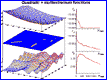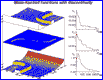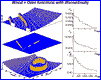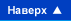© Сигма Технология 2008